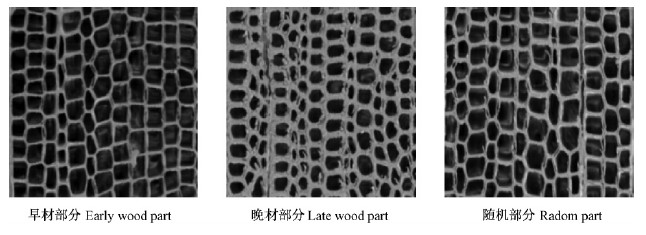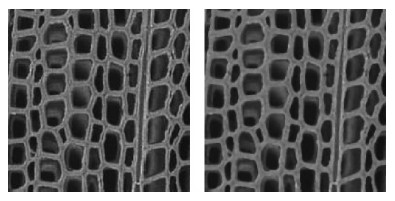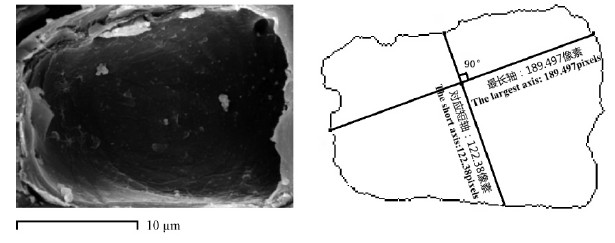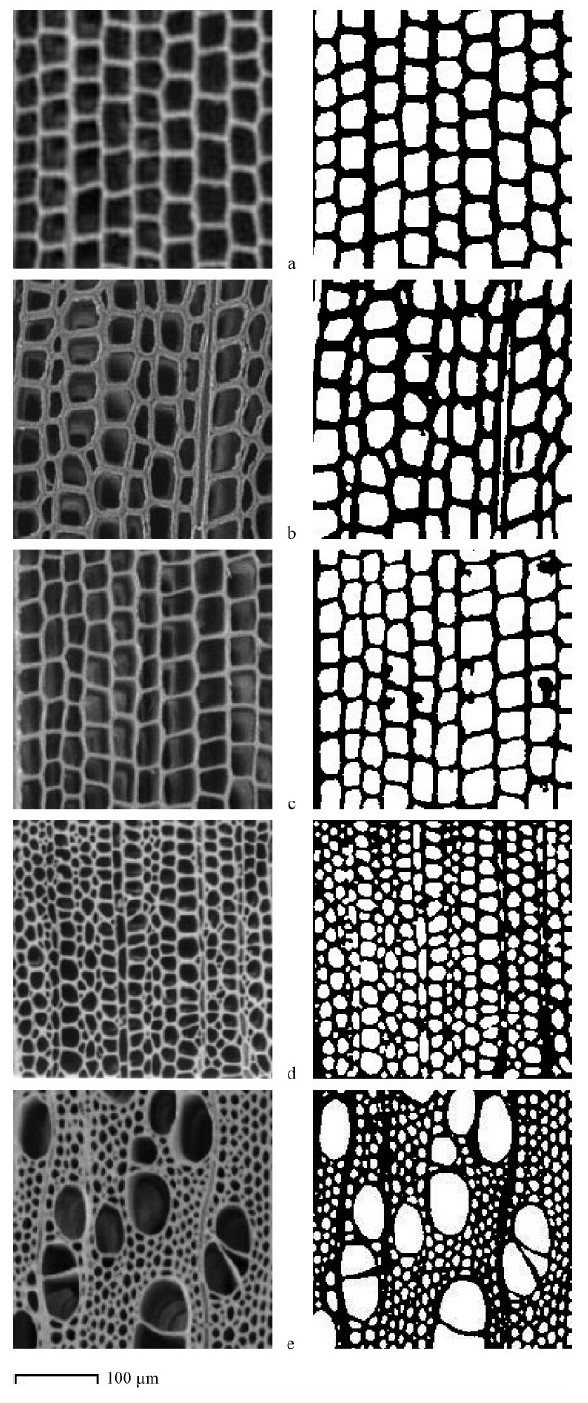﻿ 基于Graph Cuts的木材扫描电镜图像特征提取方法
 林业科学2014, Vol. 50Issue (4): 108-114PDF
DOI: 10.11707/j.1001-7488.20140416
0

#### 文章信息

Zhu Jia, Wang Hangjun

Feature Extraction Method of Wood SEM Micrographs Based on Graph Cuts

Scientia Silvae Sinicae, 2014, 50(4): 108-114.
DOI: 10.11707/j.1001-7488.20140416

### 作者相关文章

1. 浙江农林大学信息工程学院临安 311300；
2. 浙江农林大学天目学院诸暨 311800

Feature Extraction Method of Wood SEM Micrographs Based on Graph Cuts
Zhu Jia1, Wang Hangjun21. School of Information Engineering, Zhejiang A & F University Lin'an 311300;
2. Tianmu College of Zhejiang A & F University Zhuji 311800
Abstract: Generally, even the subtle differences of wood textures among different species can be clearly identified with their scanning electron microscope(SEM)micrographs. Therefore, the features extracted from the SEM micrographs can provide a reliable basis for the classification of wood micrographs. In this paper, a novel method of wood SEM micrographs was proposed for feature extraction based on Graph Cuts. Firstly, the wood SEM micrographs would be enhanced before partitioning the images into multiple segments by Graph Cuts, then extracting the contours of wood tissues and smoothing the contours of objects by mathematical morphology. Finally, nine kinds of geometric and statistical features and their computing methods were given. The experimental results on 12 wood species showed that the features we proposed had a great difference among the given wood species(also in the earlywood and latewood of each given species), which provided an important basis for the wood assortment recognition.
Key words: Graph Cuts    feature extraction    SEM    image segmentation    cell

1 材料与方法图 1 日本五针松3 种分割方式截取效果 Fig. 1 Results of Pinus parviflora under three divided ways
2 图像处理 2.1 图像增强图 2 日本榧树显微图像的图像增强效果 Fig. 2 Effect of enhancement for Torreya nucifera micrograph
2.2 基于Graph Cuts的图像分割

Graph Cuts作为一种流行的能量函数优化算法，在计算机视觉领域被普遍应用，本文采用GraphCuts算法对木材SEM显微图像进行分割。GraphCuts是一种基于图论的组合优化方法(Boykov et al.，2001a;2001b;2004)，它先将图像映射为一幅网络图，构造关于标号的能量函数后，再运用最大流/最小分割算法对网络图进行分割，得到的网络图的最小割即为目标函数的最小值。组合优化中将一个割的容量(cost)定义为非负边容量的总和，表示为:

 $\left| C \right| = \sum\limits_{e \in c} {{W_e}}。$ (1)

 $E\left( A \right) = \lambda \cdot R\left( A \right) + R\left( B \right);$ (2)
 $R\left( A \right) = \sum\limits_{p \in P} {R\left( A \right)} 。$ (3)
 $B\left( A \right) = \sum\limits_{\left\{ {p,q} \right\} \in N} {{B_{\left\{ {p,q} \right\}}}} \cdot \delta \left( {{A_p},{A_q}} \right);$ (4)
 ${B_{\left\{ {p,q} \right\}}}~\exp \left( { - \frac{{{{\left( {{I_p} - {I_q}} \right)}^2}}}{{2{\sigma ^2}}}} \right) \cdot \frac{1}{{{\rm{dist}}\left( {p,q} \right)}}。$ (5)

3 特征参数提取 3.1 边界最长轴和边界偏心率计算图 3 木材细胞边界最长轴与其短轴间的关系 Fig. 3 Relationship between D and d of the wood cell
3.2 细胞腔平均和最大面积计算

 ${A_{\rm{a}}} = \frac{A}{n};$ (6)
 $A = \sum\limits_{i = 0}^n {{A_i}} ;$ (7)
 ${A_i} = {n_i}。$ (8)图 4 各树种图像处理效果 Fig. 4 Effect of image treatment for wood species a. 鱼鳞云杉Picea jezoensis var. microsperma; b. 日本榧树Torreya nucifera; c. 金松Sciadopitys verticillata; d. 异色山黄麻Trema orientalis; e. 日本七叶树Aesculus turbinata.
3.3 壁腔比率计算

 $B = \frac{a}{A}。$ (9)

3.4 胞腔组织比计算

 $Q = \frac{A}{E}。$ (10)

3.5 细胞腔平均周长计算

 $L = \frac{P}{n};$ (11)
 $P = \sum\limits_{i = 0}^n {{P_i}}。$ (12)

3.6 平均圆度计算

 ${C_{\rm{a}}} = \frac{C}{n};$ (13)
 $C = \sum\limits_{i = 0}^n {\frac{{P_i^2}}{{4\pi {A_i}}}}。$ (14)

3.7 平均外接矩形比计算

 ${R_{\rm{a}}} = \frac{1}{n}\sum\limits_{i = 0}^n {\frac{{{A_i}}}{{{A_{ri}}}}} 。$ (15)

4 结果与分析 4.1 图像处理结果与分析

4.2 特征提取结果与分析5 结论与讨论

1)扫描电镜图像样本制备过程简单，避免了烦琐的木材切片制作工序，且SEM显微图像具有景深大、分辨率高、放大范围广等优点，有效地提高了试验数据的准备效率和精度，对于木材学的研究必将产生深远的影响，而应用SEM显微图像作为木材显微图像分类和材种识别的辅助手段将是一个重要的补充。

2)利用Graph Cuts算法对木材SEM显微图像进行分割，可以获得满意的木材细胞组织效果，且该算法具有计算速度快、精确、节省存储空间等优点。通过对分割后的图像进行观察可发现:各针叶树材的细胞形态具有一定规律，且其细胞大小和分布较为均匀;而各阔叶树材的微观构造较针叶树材更为复杂，其细胞形态不规律，细胞分布和大小也不均匀。

3)本文提出的9个特征对于木材SEM显微图像具有较好的区分能力。在给定的树种中，同一种针叶材的早、晚材以及不同种针叶材的特征参数间均存在明显的差异;而与针叶材相比，各项特征参数在同一种阔叶材的早、晚材间的差异相对不够明显，且部分特征参数间很难找到较为明显的分界值，但不同种阔叶材的各项特征参数间依然存在明显的差异。

  黄慧. 2006. 基于木材组织构造的数字图像处理系统研究. 南京: 南京林业大学硕士学位论文.(1)  刘镇波. 2004. 木材显微图像特征参数提取与树种判别方法研究. 哈尔滨: 东北林业大学硕士学位论文.(2)  马岩. 2002. 木材横断面细胞结构的数学模拟理论研究. 生物数学学报, 17(1): 64-68.(1)  任洪娥, 徐海涛. 2007. 细胞特征参数计算机的提取理论. 林业科学, 43(9): 68-73.(2)  汪杭军, 张广群, 祁亨年, 等. 2009. 木材识别方法研究综述. 浙江林学院学报, 26(6): 896-902.(1)  于海鹏, 刘一星, 张斌, 等. 2004. 应用空间灰度共生矩阵定量分析木材表面纹理特征. 林业科学, 40(6): 121-129.(1)  于海鹏, 刘一星, 孙建平. 2005. 基于小波的木材纹理分频信息提取与分析. 林业科学, 41(2): 100-105.(1)  Boykov Y, Jolly M P. 2001a. Interactive graph cuts for optimal boundary & region segmentation of objects in N-D images//Proceedings of the 8th International Conference on Computer Vision. Vancouver, Canada: IEEE Press, 1: 105-112.(1)  Boykov Y, Kolmogorov V. 2004. An experimental comparison of min-cut/max-flow algorithms for energy minimization in vision. IEEE Transactions on Pattern Analysis and Machine Intelligence, 26(9): 1124-1137.(1)  Boykov Y, Veksler O, Zabih R. 2001b. Fast approximate energy minimization via graph cuts. IEEE Transactions on Pattern Analysis and Machine Intelligence, 23(11): 1222-1239.(1)  Ford L, Fulkerson D. 1962. Flow s in networks. New Jersey: Princeton University Press.(1)  Huang T S, Yang G J, Tang G Y. 1979. A fast two-dimensional median filtering algorithm. IEEE Trans on Acoustics, Speech and Signal Process, 27(1): 13-18.(1)  Kino M, Ishida Y J, Doi M, et al. 2004. Experimental conditions for quantitative image analysis of wood cell structure, 3: Precise measurements of wall thickness. Journal of the Japan Research Society, 50(1): 1-9.(1)  Pan S, Kudo M. 2010. Segmentation of pores in wood microscopic images based on mathematical morphology with a variable structuring element. Computer and Electronic in Agriculture, 75(2): 250-260.(1)  Tou J Y, Tay Y H, Lau P Y. 2009. Rotational invariant wood species recognition through wood species verification//Nguyen N T, Nguyen H P, Grzech A. Proceedings of the 2009 First Asian Conference on Intelligent Information and Database Systems(ACⅡDS), The Institute of Electrical and Electronics Engineers, Inc, Dong Hoi, 115-120.(1)  Tukey J W. 1971. Exploratory data analysis. Addison-Wesley, Reading, MA.  Wang H, Qi H, Li W, et al.2009. A GA-based automatic pore segmentation algorithm//Proceedings of the First ACM/SIGEVO Summit on Genetic and Evolutionary Computation(Shanghai, China, June 12-14, 2009). GEC' 09, ACM, NY, 985-988.(1)  Xu F. 2008. Anatomical Figures for Wood Identification. Beijing: Chemical Industry Press.(1)